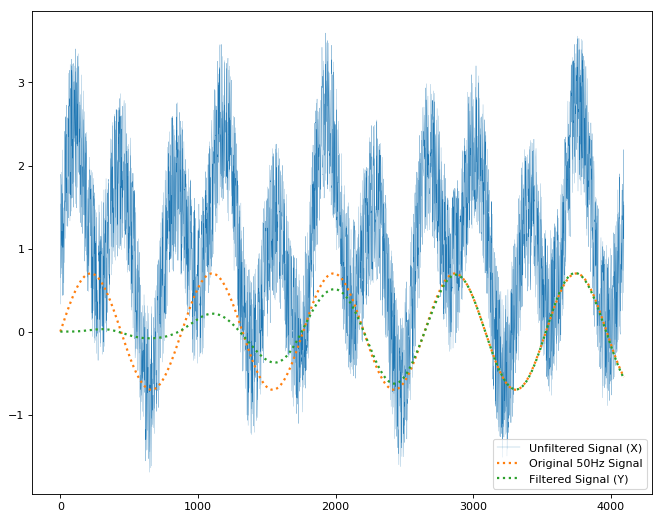Scientific/Signal API# Scientific/Signal API

## Class: LinearFilter

New in SDK 3.0

A LinearFilter represents any Finite Impulse Response (FIR) or Infinite Impulse Response (IIR) linear filter. It provides a convenient mechanism for keeping track of the entire configuration and state for such a filter, but is just a convenience: It contains no hidden state, and could be reconstructed every `update` from its constituent fields, if desired.

The filter is represented in transfer function form, as arrays of numerator and denominator coefficients. The filter is realized in direct form II, requiring only a single array of delay elements with length `max(b.length, a.length) - 1`.

For the purposes of local design and debugging, the following should be equivalent:

##### JavaScript
``````import { LinearFilter } from "scientific/signal";
var filt = new LinearFilter(b, a, zi);
var y = filt.update(x);
var zf = filt.z;
``````
##### Python
``````y, zf = scipy.signal.lfilter(b, a, x, zi)
``````

### Example

As a simple example, a butterworth bandpass filter can be constructed as follows:

``````import { LinearFilter } from "scientific/signal";
``````

#### Generate some sample data

Typically this would come from an accelerometer, gyro, or other sensor.

``````const numSamples = 4096;
const sampleFreq = 44100;
const X = new Float32Array(numSamples);
for (let i = 0; i < X.length; i++) {
const t = i / sampleFreq;
// Form a signal containing a 50 Hz sinusoid of amplitude 0.7
var S = 0.7 * Math.sin(2 * Math.PI * 50 * t);
// ...and a 120 Hz sinusoid of amplitude 1.
S += Math.sin(2 * Math.PI * 120 * t);
// Corrupt the signal with zero-mean white noise with a variance of 4.
X[i] = S + 2 * Math.random()
}
``````

#### Construct a filter

Typically this would be done at application startup, and the same filter(s) reused for the duration of application execution.

``````const filter50hz = new LinearFilter(
new Float32Array([2.88394643e-09, 0.0, -8.65183928e-09, 0.0, 8.65183928e-09, 0.0, -2.88394643e-09]),
new Float32Array([1., -5.99415495, 14.97093756, -19.94220015, 14.94252458, -5.97142422, 0.99431717]));
``````

`b` and `a` filter coefficients were calculated using `scipy.signal`

##### Python
``````nyquist = 0.5 * sampleFreq
b, a = scipy.signal.butter(3, [40 / nyquist, 60 / nyquist], btype='band')
``````

#### Update the filter

Typically this would be done each time new sensor samples come in:

``````const y = filter50hz.update(X);
``````

`y` contains the frequency components of `X` between 40hz and 60hz, with the 120Hz signal and noise filtered out.See the example Scientific app on Github

#### Properties

`Float32Array`

`a` represents a copy of the coefficients of the polynomial describing the denominator in the form:

``````a + az^-1 + ... + a[N]z^-N
``````

If `a` is not `1`, both `a` and `b` are normalized by `a`.

`Float32Array`

`b` represents a copy of the coefficients of the polynomial describing the numerator in the form:

``````b + bz^-1 + ... + b[M]z^-M
``````

`Float32Array`

`z` represents a copy of the internal state of the filter delay elements. If not provided, defaults to an array of zeros.

#### Methods

##### update()

`update(x: Float32Array)`

Returns: `Float32Array`

`update` runs each of `x`'s data points through the filter, updating the internal state `z` at each iteration, and returning the filter output as a `Float32Array`.